3D Geometry Primer: Chapter 1 - Issue 02 - Vector Arithmetic by (21 August 2000) Return to The Archives
 Vector ArithmeticIn this issue, we're going to learn how to calculate with vectors: how to add or subtract vectors, how to multiply vectors with scalars or other vectors, etc...Normally, we work with free vectors only, because most applications don’t care about the starting point of vectors. You can represent e.g. the speed of 2 points of a car with 2 bound vectors. But all points of that car have the same speed (it would be a very unpleasant feeling if the back wheels had twice the speed of the front wheels). So there’s no need to represent both speeds with 2 different bound vectors. Because they store the same information (same direction, sense and magnitude), you can represent both speeds with 1 free vector, which we can represent in the origin.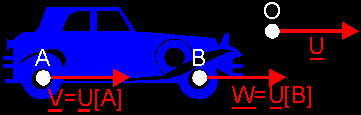So, from now, if we speak about vectors, we always talk about free vectors. Except if we mention otherwise.Just like real numbers, you can add vectors, and it’s also possible to multiply them. However there are 3 kinds of multiplications! The first, a scalar product multiplies a vector with a real value and results in a vector. The 2nd is the dot product and multiplies 2 vectors with a real value as the result. And the last multiplication is the cross product which turns 2 vectors back into a new vector.

 (I) Negative Of A Vector: -VTo get the negative of a vector, just switch the sense of the vector. When you do this twice, you get back the original vector: –(–V)=V You can also say "to get the negative, swap tail and head": –AB=BA(II) Scalar Product: rV or r*VIt is possible to multiply a vector V with a real number r. To do that, just multiply the magnitude (which is a real number too) with r.Notice that the magnitude of a vector needs to stay positive, so if you scale V with a negative value r, then you need to mulitply the magnitude with abs(r) and switch the sense of the vector: if r<0 then r*V=abs(r)*(–V).Example 1: –2V = –2*V = 2*(–V) = –(2*V). Example 2: –1V = –1*V = –V (just what we expected)Of course, if you scale V with the value 0, then you get a null vector.Normalizing: if you scale a vector V with 1/||V||, then you create a vector with magnitude 1. Because you divide its magnitude by its magnitude. This method of creating unit vectors is called normalizing. You can write it down like this: V1=V/||V|| with V1 being the derived unit vector.

 (III) Vector Addition: U+VTo calculate the sum of two vectors, you can use two methods:1. Lay the 2nd vector V with its tail at the head of the 1st vector U, and draw the sum U+V between the tail of U and the head of V. 2. Lay both vectors U and V with their tails together in the origin O and draw a parallelogram. Now draw the sum between the origin O and the opposite corner of the parallelogram.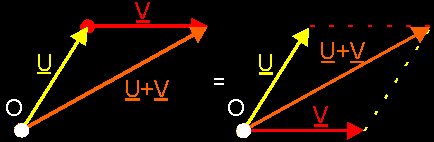An example? Say, you're in a little boat and you're rowing and rowing with a force R, trying to reach the shore. But, meanwhile, the water pulls your rowing boat downstream with a force W. You approach a giant waterfall. Then, the real force your boat is subjected to is the sum R+W, and you will go in that direction. The only thing that you can hope for, is that you reach the shore first, and not the waterfall.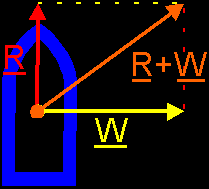If you need to sum more vectors (e.g. U+V+W), you can use those two methods again:1. Lay all vectors with their heads and tails together and draw the sum between the start and the end. 2. First add 2 vectors with a parallelogram: U+V. Then add W to this sum: U+V+W = (U+V)+W.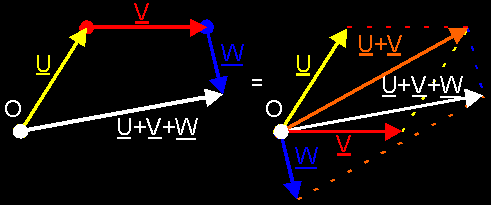Notice that it doesn't matter in witch sequence you add the vectors: (U+V)+W = (V+U)+W = U+(V+W) = V+(U+W)

 (IV) Vector Subtraction: U-VAgain 2 methods: 1. Scale vector V with –1. You get –V = –1*V. Then add –V to U: U+(–V) 2. Question yourself with vector you need to add to V, to get U as result. (This is for professionals only! :)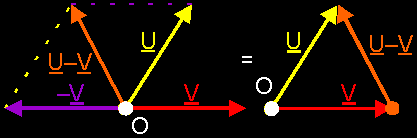(V) Dot Product: U·VIf two vectors U and V (with magnitudes respective ||U|| and ||V|| ) enclose an angle theta, then the dot product of U and V is given by U·V=||U||*||V||*cos(theta). Notice that at the left there's a product between vectors, and at the right there are only products between real values. So, the dot product of 2 vectors result in a real value!Notice also that: ° U·V=V·U. ° Use the dot product to calculate an angle between 2 vectors. If you divide the dot product by the magnitudes of both vectors, then the only thing that remains is: (U·V)/(||U||*||V||)=cos(theta). You have found the cosine of the angle between those vectors. Then, you can use an inverted cosine function to find the actual angle. Unfortunately, you won't be able to use this method, since you don't know how to calculate U·V in a different way. You will have to wait till issue 6 to do that.° What means V·V? Because the angle theta "between V and V" is zero, cos(theta)=1, so V·V=||V||.||V||. Like with real values we can also say: V˛=||V||˛. (Remember this: if you need to say something about the magnitude of a vector, try to say something about ||V||˛ so that you can use the dot product V·V.) ° What means 0·V? Because you can't define an angle between the null vector and another vector, we define 0·V as equal to zero because the length of 0 is zero. ° What means U·V=0? The product ||U||*||V||*cos(theta) consists of 3 values. If the product is equal to zero, this means that at least 1 of these 3 values is zero. If ||U||=0 or ||V||=0, then you have a null vector in the product. If cos(theta) is 0, this means that theta is 90° or -90°. In that case U and V stand perpendicular to each other. This also means that the null vector is perpendicular to every vector, because V·0=0 for each vector V.

 (VI) Cross Product: U×VThe cross product U×V is a vector given by the following definition: 1. The direction of U×V is perpendicular to both U and V. 2. Its sense is given by the rule of the corkscrew: If you turn your fingers from U to V, then the sense of U×V is given by your thumb. In right-handed systems, this has to be done with you right hand. (Normally we always use right-handed systems. We’ll talk about this later on.) 3. Its magnitude ||U×V|| is given by the area of the parallelogram given by vectors U and V. This area is equal to ||U||*||V||*sin(theta) with theta the angle enclosed between vectors U and V.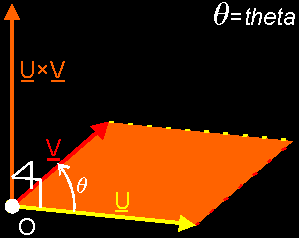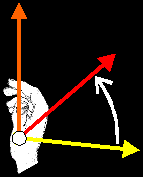Notice that: ° U×V is perpendicular to both U and V. In the future, this will be very, *very*, *VERY* important! ° U×V is not the same as V×U . In fact: U×V = –V×U . ° What is V×V? There are more than one vectors that are perpendicular to "V and V". We define V×V=0 because "the area between V and V" is 0 . ° What is 0×V? Because each vector stand perpendicular to 0, you can find more than one vector that is perpendicular to 0 and V. We define 0×V=0=V×0, because "the area between 0 and V" is 0 (||0||=0) . ° What means U×V=0? U×V=0 if ||U×V||=0. This means that the product ||U||*||V||*sin(theta)=0 . This product is 0 if ||U|| or ||V|| is 0 (in case of a null vector) or if sin(theta)=0. In that case theta is 0° or 180°. This means that U and V have the same direction. ° Similar to the dot product, if you divide the cross product by the magnitudes of both vectors, you get the sine of the angle between U and V: (U×V)/(||U||*||V||)=sin(theta). You shouldn't use this to find the angle itself, because... 1. it's much slower than using the dot product way and 2. the angles theta and (180°–theta) have the same sine value! If you used an inverted sine function to find the angle between 2 vectors, you wouldn't be able to distinguish between e.g. 30° and (180°-30°)=150°, which is definitely a different situation!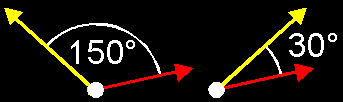OK, that's it for today. Next time, we will continue with our vector arithmetic, but first a little exercise =] :Given below is the triangle ABC, and the point D is in the middle of points AB (so the distance between A and D is equal to the one between D and B, hint :). You only know the vectors CD and DB. I want you to express the following vectors as a combination (addition, subtraction, negation, multiplication) of those 2 vectors: CB, AD, DA, AB and CA. You can use free vectors. So, get started with the puzzle and I'll see you next week.Regards, Bramz